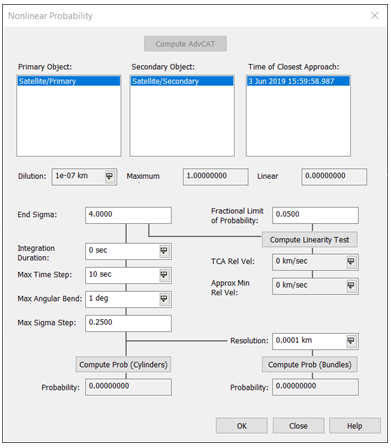Back

# There is a New Lesson in Town

Are you into determining if two satellites might collide? Well, we have a new lesson for you! The lesson is “Advanced CAT Tool: Assessing the Threat of a Collision”. There are other tutorials that use the Advanced CAT Tool, however this lesson is unique and focuses on the following:

• Analytic
• Numeric
• Patera 2005
• Maha

The Advanced CAT tool provides a convenient way for you to carry out close approach analyses for multiple satellites and two-line element (TLE) sets or your own data. The Nonlinear Probability tool can be used to compute conjunction probability for nonlinear relative motion.

In the tutorial, a primary satellite (your satellite for instance) is prebuilt and saved as an ephemeris file. The data in the ephemeris file contains covariance data. You can generate a STK *.e file with HPOP using a frame defined by a Conjunction Data Message (CDM). The CDM specification allows many frames, but having all the data in *.e files where the frame is specified in the metadata allows for the appropriate comparisons. The secondary satellite is built using HPOP and you manually enter orbital parameters, force models, and covariance. Force models can be used to define a precise representation of a satellite's force environment for use in HPOP analysis. HPOP is capable of propagating the state error covariance matrix as it is propagating ephemeris.

Using the Advanced CAT tool, you will complete a linear and a non-linear analysis. In our Level 2 – Advanced training, there is an introduction lesson called “Assessing the Threat of a Collision” where you use two-line mean element (TLE) sets to perform a linear analysis. What separates this lesson is propagating satellites (HPOP) using your data and choosing a collision probability analysis type by creating a custom report using the following:

 Collision Probability Summary Analytic The probability of collision computed using an analytic method based upon Ken Chan's paper. Numeric The probability of collision computed using a numeric method based upon Sal Alfano's paper. Patera 2005 The probability of collision computed using an analytic method based upon Russell Patera's paper that appeared in the J. of Guidance, Control and Dynamics, vol. 28 (6), 2005. Maha The probability of collision computed based on the Mahalanobis distance method.

The first analysis is done using a fixed dimension (threat volume) which specifies the dimensions of the threat volume ellipsoid on the basis of values you enter. Next, you switch to a covariance dimension which uses the position covariance associated with the object to size and orient the threat volume ellipsoid. This is when you are introduced to the Nonlinear Probability tool.The Nonlinear Probability tool allows you to test for linear relative motion and provides two methods for computing conjunction probability: adjoining parallelepipeds (bundles) and adjoining tubes (cylinders).The lesson breaks the tool out in detail, explaining each field and value and when to choose Cylinders or Bundles in your analysis.

This is a very technical lesson, but informative, and written in a way that anyone new to the world of satellites and STK can learn something valuable. In all honesty, it’s simply a fun lesson!

#### Conjunction Analysis

Detect and assess potential collisions in space.